Follow

# How are the Total at Completion and Cost Variance fields on the Costs entity calculated?

### Default Cost Entity Fields:

PPO’s default configuration includes the following cost entity fields:

• Budget – This field allows the Project Manager to record the project budget that has been approved.
• Actual / Spent – This field allows the Project Manager to record the money that has been spent on the project (in some cases client’s rename this field to Invoiced).
• Committed – This field allows the Project Manager to record the money that has been committed and not yet spent on the project. This is typically used when a purchase order has been generated but an invoice has not been generated yet.
• Estimated to Complete – This is sometimes renamed Forecast and should be used by the Project Manager to forecast / estimate the amount of money that he/she is still going to use to complete the project.
• Total at Completion – This is a calculated field and is calculated as follows: Actual / Spent + Estimate to Complete (it thus takes the money already spent on the project plus the amount still required to complete the project and provides a calculated total of what the spend will be at the end of the project).
• Cost Variance – This is a calculated field and is calculated as follows: Budget – Total at Completion (it thus determines whether a project will under or over spend by looking at the budget vs. the calculated total at completion).

To understand the working of these fields the following example has been provided: The “Workflow Technology Project” has a total budget of R 100,000 which is made up of CAPEX and Labour and the duration of the project is two months.

### Step 1 - Load Initial Budget

The Project Manager can record his/her project budget in PPO’s Costs entity as follows: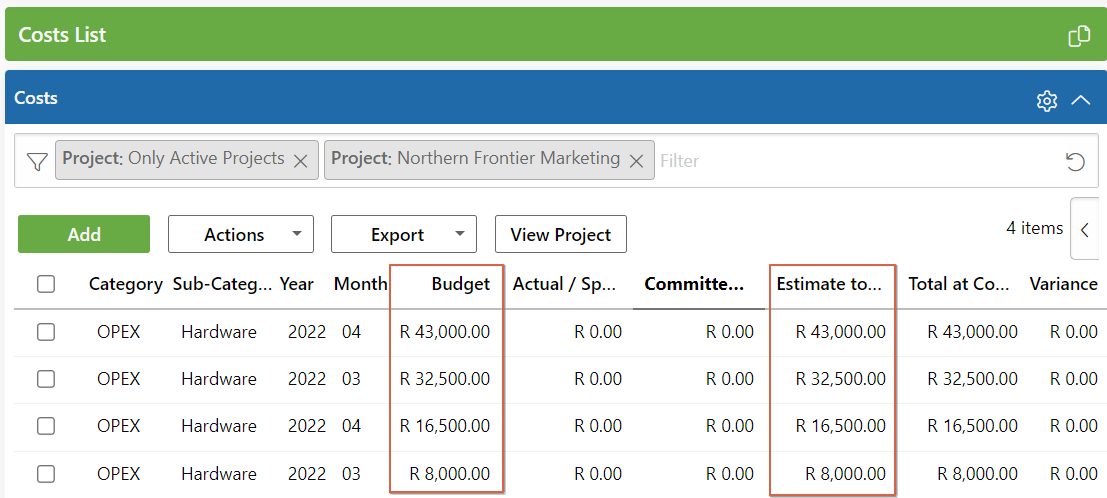Note: when the project starts, the Budget and the Estimate to Complete (Forecast) should typically be the same.

The project cost reporting will be as follows: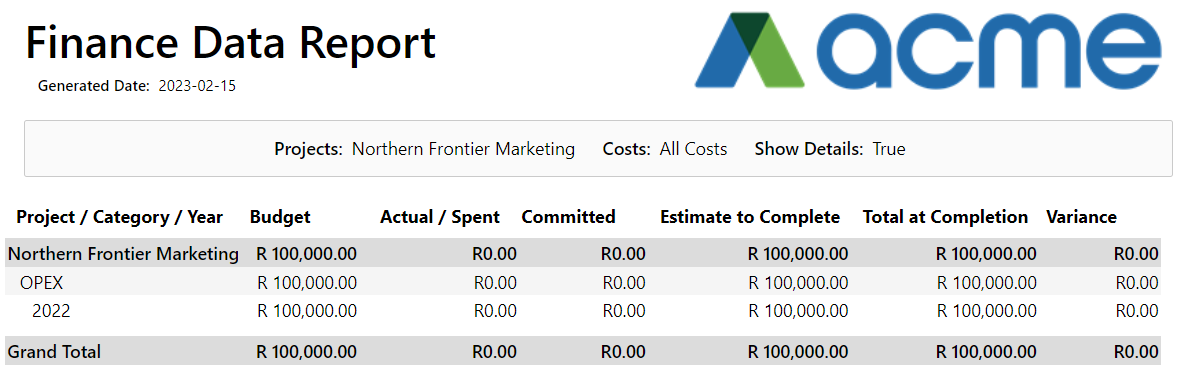The calculated fields are thus:
Total at Completion = Spent (R 0) + Committed (R 0) + Estimate to Complete (R 100,000) = R 100,000.
Cost Variance = Budget (R 100,000) – Total at Completion (R 100,000) = R 0.

### Step 2 - Load Project Actual / Spend & Adjust Estimate to Complete / Forecast:

At the end of the first month / reporting cycle the Project Manager can update the project costs. Typically the spend for the period will be recorded along with any committed spend and the estimate to complete will be modified. In our example the Actual / Spent & Committed for March for both categories has been updated, the Budget has not been changed and the Estimate to Complete for March has been updated to R0.00 because we are not planning to spend any more money in March.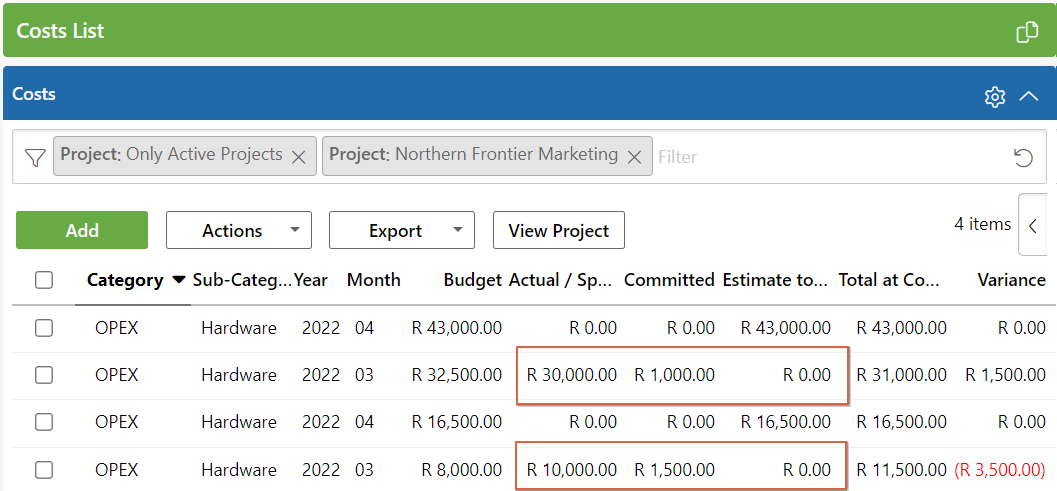The project cost reporting will be the following at this stage: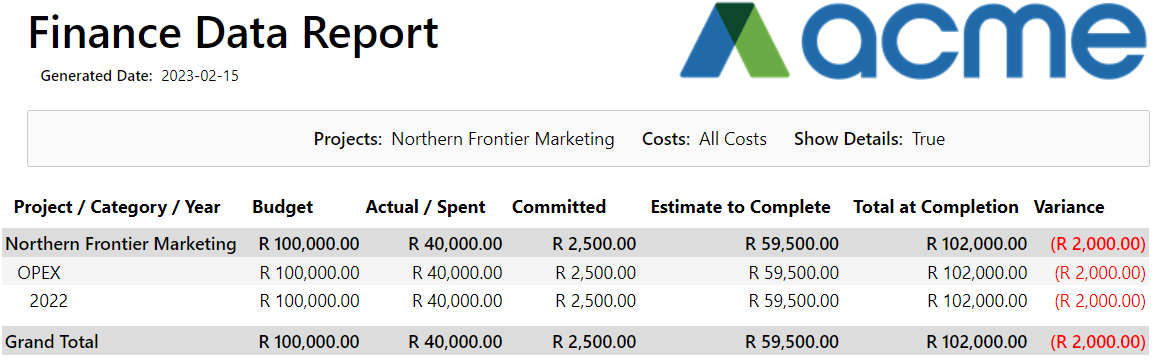You will notice that the project now has a projected overspend of R 2,000 and you can see that there is an overspend on Opex for R 2,000. Because we are using the Estimate to Complete / Forecast we are able to show the users of the potential overspend before it occurs. Without using that field the total budget would have been R 100,000 and a total spend of R 40,000 would show the users that there is still R 60,000 left on the budget but you would not have seen the potential overspend.

The calculated fields are thus:
Total at Completion = Actual / Spent (R 40,000) + Committed (R 2,500) + Estimate to Complete (R 59,500) = R 102,000.
Cost Variance = Budget (R 100,000) – Total at Completion (R 102,000) = -R 2,000.

### Step 3 - Final Project Costing at Completion:

At the end of the project the Estimate to Complete & Committed field should be R 0.00 and the final Actual / Spent should be recorded as follows: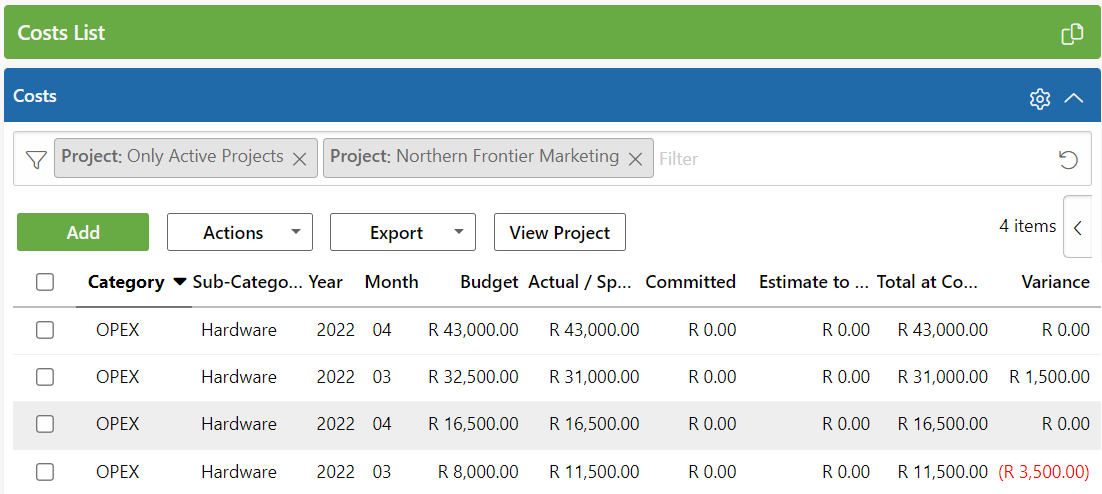The final project cost report will thus be: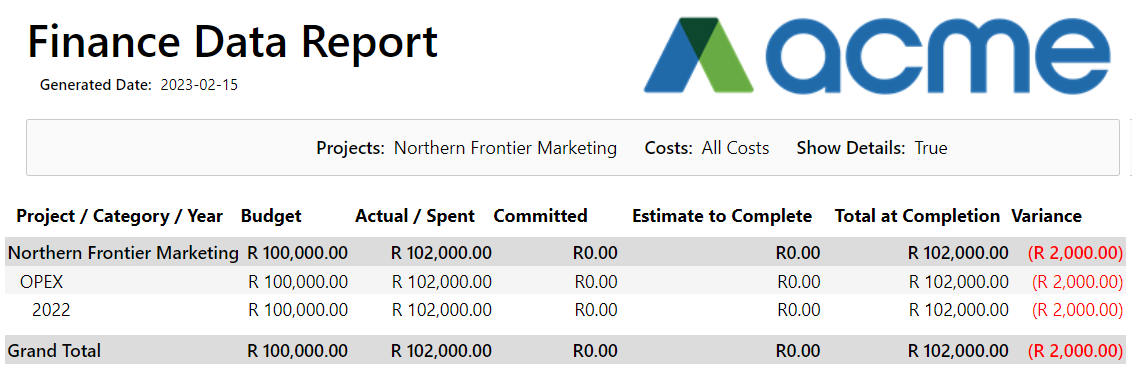The calculated fields are thus:

Total at Completion = Actual / Spent (R 102,000) + Committed (R 0) Estimate to Complete (R 0) = R 102,000.
Cost Variance = Budget (R 100,000) – Total at Completion (R 102,000) = -R 2,000.